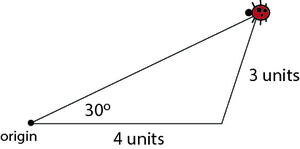# A task related to standard 7.G.A.2

Alignments to Content Standards: 7.G.A.2

Starting at the origin, a ladybug walked 4 units east. Then she walked a distance of 3 units in an unknown direction. At that time she was 30 degrees to the north of her original walking direction.

The diagram shows one possibility for the ladybug’s final location. Find a different final location that is also consistent with the given information, and draw the ladybug there.## IM Commentary

When students successfully complete this task, they will have shown that there is more than one triangle with a 30-degree angle adjacent to a side of length 4 units and opposite to a side of length 3 units. This task can be part of a more general study directed at "noticing when" "three measures of angles or sides" "determine a unique triangle, more than one triangle, or no triangle." (7.G.A.2)

In this case, the three given measures determine more than one triangle. (Thus in high school, there will be no "angle-side-side theorem" analogous to the side-angle-side and similar theorems.)

From a standpoint of geometric precision, it is best for the student to use a compass to find (or verify) the second possible location. Put the point of the compass at the lower-right vertex of the triangle, put the pencil point at the upper-right vertex, and construct a circle of radius 3 units that intersects the long leg of the triangle at the desired point.

## Solution

The diagram below shows the second possible location for the ladybug.Like the first diagram, this one shows a ladybug that has walked 4 units east from the origin point, and then a distance of 3 more units, ending at a point 30 degrees north of east. The diagram shows that there are two non-congruent triangles with the three given measures.